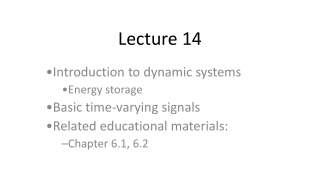DownloadDownload PresentationLecture 14

# Lecture 14

Download Presentation## Lecture 14

- - - - - - - - - - - - - - - - - - - - - - - - - - - E N D - - - - - - - - - - - - - - - - - - - - - - - - - - -
##### Presentation Transcript

1. Lecture 14 Introduction to dynamic systems Energy storage Basic time-varying signals Related educational materials: Chapter 6.1, 6.2

2. Review and Background • Our circuits have not contained any energy storage elements • Resistors dissipate energy • Governing equations are algebraic, the system responds instantaneously to changes

3. Example: Inverting voltage amplifier • The system output at some time depends only on the input at that time • Example: If the input changes suddenly, the output changes suddenly

4. Inverting voltage amplifier – switched response • Input and response:

5. Dynamic Systems • We now consider circuits containing energy storage elements • Capacitors and inductors store energy • The circuits are dynamic systems • They are governed by differential equations • Physically, they are performing integrations • If we apply a time-varying input to the system, the output may not have the same “shape” as the input • The system output depends upon the state of the system at previous times

6. Dynamic System – example • Heating a frying pan

7. Dynamic System Example – continued • The rate at which the temperature can respond is dictated by the body’s mass and material properties • The heat out of the mass is governed by the difference in temperature between the body and the surroundings: • The mass is storing heat as temperature

8. Dynamic System Example – continued

9. Time-varying signals • We now have to account for changes in the system response with time • Previously, our analyses could be viewed as being independent of time • The system inputs and outputs will become functions of time • Generically referred to a signals • We need to introduce the basic time-varying signals we will be using

10. Basic Time-Varying Signals • In this class, we will restrict our attention to a few basic types of signals: • Step functions • Exponential functions • Sinusoidal functions • Sinusoidal functions will be used extensively later; we will introduce them at that time

11. Step Functions • The unit step function is defined as: • Circuit to generate the signal:

12. Scaled and shifted step functions • Scaling • Multiply by a constant • Shifting • Moving in time

13. Example 1 • Sketch 5u0(t-3)

14. Example 2 • Represent v(t) in the circuit below in terms of step functions

15. Example 3 • Represent the function as a single • function defined over -<t<.

16. Exponential Functions • An exponential function is defined by •  is the time constant •  > 0

17. Exponential Functions – continued • Our exponential functions will generally be limited to t≥0: • or: • Note: f(t) decreases by 63.2% every  seconds

18. Effect of varying 

19. Exponential Functions – continued • Why are exponential functions important? • They are the form of the solutions to ordinary, linear differential equations with constant coefficients